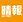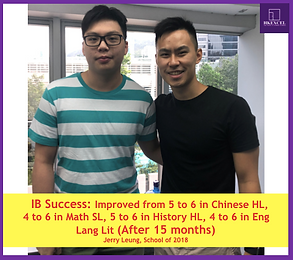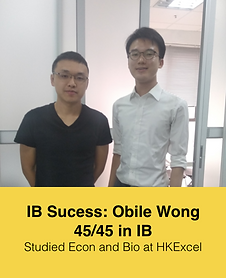top of page
###### Recognised by:# IB MATH AI vs AA

IB math AA (Analysis and Approaches) or IB Math AI (Application and Interpretation) are totally different subjects. If you choose the wrong course, you could put your future at stake. In the old curriculum (last exam 2020 November), it was very easy to decide which math level to choose; if you are weak at math, then go for Math Studies at Standard Level (SL). If you wanted to go for a science degree, you could choose IB Math SL. For math-intensive degrees like physics and engineering, taking IB Higher Level (HL) math was intuitively a must.

With the introduction of the new IB syllabus in 2021, we now have two courses - IB Math AA and Math AI, each available at both SL and HL levels. The inaugural exams for these subjects were in May 2021, and the curriculum is set to run for a decade, culminating in 2028. The IBO will then reevaluate and restructure the syllabus. So, the question remains: which one should you choose? Our IB math specialist at HKExcel will reveal EVERYTHING you need to know in this article.

## The Difference between IB Math AA & AI

To understand the differences between IB Math AA and IB Math AI, it is important to note their different focuses. IB Math AA emphasizes algebra, problem-solving, calculus, and pure mathematics. This curriculum features abstract and challenging exam questions. On the other hand, IB Math AI includes topics not found in IB Math AA, such as Voronoi diagrams, statistics and probability (like Poisson distribution), and matrices.

IB Math AA follows a classical, conceptual, and abstract learning approach, delving into advanced calculus, integration, and pure mathematics. It offers both SL and HL options. This course is suitable for students interested in abstract theories and proofs, and who are passionate about generalizations.

IB Math AI, on the other hand, requires a practical perspective, focusing on the application of mathematical concepts. It covers statistics, probability, modeling, and more. Like Math AA, it provides Standard and Higher Level difficulty options. Students keen on applying math in real-world contexts may find IB Math AI a more fitting choice.

It is incorrect to deem one curriculum as more challenging than the other, as they are different subjects akin to subjects like biology and chemistry. Students should consider their interests and aspirations if they are deciding between IB Math AA and IB Math AI.

## SL VS HL - Comparing the Two Levels in IB Math AA & AI

When it comes to IB Math, both AA (Analysis and Approaches) and AI (Applications and Interpretation) have two levels: Standard Level (SL) and Higher Level (HL). So, what are the main differences?

### Time and Examinations:

• Both IB Math AA and AI demand 150 hours for SL courses and 240 hours for HL courses.

• SL students will tackle two exams, while HL students prepare for three.

### Depth of Study:

• The SL curriculum introduces fewer topics than the HL. For example, while IB Math AI SL covers 13 chapters, its HL counterpart delves into 16.

• Some topics in IB Math AA SL are also present in IB Math AA HL.

• Despite their differences, AA and AI share five core areas: number and algebra, functions, geometry and trigonometry, statics and probability, and calculus. The emphasis varies between them.

### Course Specifics:

• IB Math AA:

• HL: This aligns with the earlier IB Math HL and places a strong emphasis on theoretical mathematics, particularly calculus.

• SL: Closer to the previous Math SL, it provides an introduction to calculus, albeit less intensively than AAHL.

• IB Math AI:

• HL: Positioned between the former Math HL and Math SL in terms of complexity, IB Math AI HL includes topics not previously covered in the older IB Math HL curriculum.

• SL: An evolution of the older Math Studies, IB Math AI SL presents new mathematical topics for exploration.

Ultimately, the decision between choosing SL and HL for your IB Math AI or AA courses depends not only on time commitment and examination structure but also on your specific preferences of mathematical areas and topics.

## Detailed Breakdown of Topics & Chapters in IB Math AA and AI Courses

If you are looking for a detailed breakdown of the topics and chapters in IB Math AA and IB Math AI, our IB math expert has created videos to guide you through the curriculum. Explore the videos below for a comprehensive overview:

## How to choose IB Math AA or AI?

Having learned the differences between IB Math AA and AI, and now possessing a general understanding of the topics covered in the syllabus, you may start to wonder how to choose between them. There are many factors you should consider, as IB Math is definitely a top priority in your IB journey. Universities often have specific math requirements for enrollment, and it also impacts your future career building.

### University or College Requirement

B Math is always the NUMBER ONE subject that you need to think of in your IB journey, as most universities require specific math levels and subjects as minimum requirements for enrolment, especially for disciplines requiring proficient mathematical skill. IB students have to choose wisely and carefully.

Discipline: Engineering, Physics, Computer
IB Math requirement: IB Math AA HL

For science or economics students, you will be required to take IB Math HL, as these disciplines demand strong analytical skills and prefer students with a strong logical mind. Imagine one day you become the finance head of a company or a research specialist in a medical engineering firm; you will need to equip yourself with good knowledge of statistics, applied math, and analysis based on calculus.

Discipline: Law, General Business, Arts, Language, Social Science

IB Math requirement: No specific math requirement

For IB students who are good at languages and words, there is no need to take IB Math AA or AI HL, as these subjects are not required in the discipline. However, competitive degree programs such as the Bachelor of Law require students to score at least 38/45 or above in the IB diploma to be considered for admission to top universities in Hong Kong, UK, US, Australia, Canada, etc. For example, the London School of Economics requires a minimum IB score of 38/45 for their Law program, with HL scores of 7, 6, 6. Thus, pick a math level that you are confident you can get good results in.

### Future Career Paths

When considering your future career path after your IB journey, IB Math AA offers a focus on theoretical mathematics, including topics like calculus and integration. This makes it an ideal choice for students interested in math-intensive degrees like engineering, physics, computer science, or pure math. IB Math AA provides a strong foundation in mathematical theories and concepts, laying the groundwork for future mathematical pursuits.

On the other hand, IB Math AI emphasizes the practical applications of math, tackling real-life problems and statistics. It proves invaluable for students interested in careers in the humanities, statistics, business, design, psychology, or specific fields of economics. IB Math AI equips students with abstract mathematical knowledge, enabling them to apply mathematical concepts to various real-world scenarios.If you want to ace IB Math AA or IB Math AI, please ! We hope to be part of your IB journey and help you ace your IB Math AA/AI exam!

How will our IB Math tutor help you in the free trial?

- Our IB Math tutor will help you master the topics in IB Math AA and AI that you find most challenging

- You will learn the most updated and effective IB Math AA and AI exam skills related to the topic

- You will master all the IB Math AA and AI exam questions that can show up for the topic

- You will receive a copy of our complete, concise and exam-based IB Math notes for the topic

- You will receive a copy of our IB Math Questionbank, including all question types that can show up for the topic

FREE trial IB Math classes are available in our centers or online through Skype!

Fill in the form below to book your IB Math FREE trial now!

Available subjects:
IB Math Analysis SL & HL, IB Math Application SL & HL, IB Math HL, IB Math SL, IB Math Studies

HKExcel Locations:

(Hong Kong) Room 702, Honest Building, 9-11 Leighton Road, Causeway Bay, Hong Kong
(Kowloon) Island Centre, 470 Reclamation Street, Mong Kok

Thanks! Message sent.

If you have questions about the free trial lesson, or need assistance with the registration, please call us at +852 2663 9194 or message us at +852 6365 7481 via WhatsApp.bottom of page